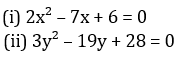Lok Sabha Election 2019 | Quantitative Aptitude Quiz

Dear Aspirants,

Quantitative Aptitude Quiz For SBI PO/Clerk Prelims

Numerical Ability or Quantitative Aptitude Section has given heebie-jeebies to the aspirants when they appear for a banking examination. As the level of every other section is only getting complex and convoluted, there is no doubt that this section, too, makes your blood run cold. The questions asked in this section are calculative and very time-consuming. But once dealt with proper strategy, speed, and accuracy, this section can get you the maximum marks in the examination. Following is the Quantitative Aptitude quiz to help you practice with the best of latest pattern questions.

ATTEMPT IN HINDI

Directions (1-5): The following table shows the percentage break up of total votes obtained by four different
parties from three different states. Study the table carefully to answer the questions given below.

Note: Total votes in different states are also associated with them. (in crors)Q1. Total votes obtained by BJP in UP is how much more than the total votes obtained by same party in Maharashtra? (in crore)

3.75
4.75
8.75
6.75
4.25

Q2. What is the average no. of votes obtained by all parties in MP?

2.5 cr
1.5 cr
2 cr
3.5 cr
4 cr

Q3. What is the ratio of votes obtained by INC in UP & Maharashtra together to the total votes obtained by TMC in same cities together?

19 : 17
17 : 19
17 : 13
13 : 17
21 : 23

Q4. If 40% votes are invalid in votes obtained by BJP in Maharashtra, then valid votes obtained by BJP in Maharashtra is

18 cr
14 cr
10 cr
16 cr
12 cr

Q5. Find the total no. of votes obtained by all parties, except BJP given in the table in UP & MP together.

4.25 cr
10.25 cr
8 cr
8.25 cr
6.25 cr

Q6. Two busses start from Delhi to Karnal at 8 am and 10 am respectively. Speed of slower bus and faster bus is 20 m/sec and 25 m/sec respectively. If distance between Delhi to Karnal is 200 km then at what time faster bus will catch the slower bus?

8h
10 h
12 h
6 h
14 h

Q7. A man can row 7 km/hr in still water. If the river is running at 3 km/hr, it takes 6 hours more in upstream than to go downstream for the same distance. How far is the place?

48 km
36 km
42 km
40 km
30 km

Q8. A boat running downstream covers a distance of 16 km in 2 hours while for covering the same distance upstream, it takes 4 hours. What is the speed of the boat in still water?

4 kmph
6 kmph
8 kmph
12 kmph
10 kmph

Q9. A man can row at 5 kmph in still water. If the velocity of current is 1 kmph and it takes him 1 hour to row to a place and come back, how far is the place?

2.4 km
2.5 km
3 km
3.6 km
4.6 km

Q10. A boat takes 9 hr to travel a distance upstream and takes 3 hr to travel the same distance downstream. If the speed of the boat in still water is 4 kmph, then what is the velocity of the stream?

4 kmph
3 kmph
6 kmph
2 kmph
8 kmph

Directions (11-15): In each of these questions, two equations (I) and (II) are given. You have to solve both the equations and give answer

Q11.if x > y
if x ≥ y
if x < y
if x ≤ y
if x = y or no relation can be established between x and y.
if x > y
if x ≥ y
if x < y
if x ≤ y
if x = y or no relation can be established between x and y.
if x > y
if x ≥ y
if x < y
if x ≤ y
if x = y or no relation can be established between x and y.
if x > y
if x ≥ y
if x < y
if x ≤ y
if x = y or no relation can be established between x and y.
if x > y
if x ≥ y
if x < y
if x ≤ y
if x = y or no relation can be established between x and y.

You May also like to Read: